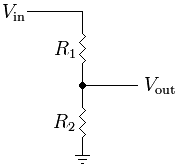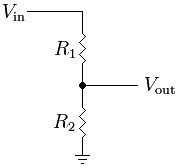# Low-pass filters, frequency selection and H(jw)

• Bassalisk
In summary, the author is not comfortable with the idea of low pass filters and discusses how these filters work and how they can be used. He sketches out three examples of simple low pass RC filters and calculates their reactance values. He then applies a voltage divider to find the cutoff frequency for each filter. Lastly, he discusses how resonant filters work and how they can be tuned.f

#### Bassalisk

Hello,

Recently I've encountered with this topic. Low pass filters and such.

But, generally I am not comfortable with this new idea.

How does this frequency selection works? I mean, I know the math behind it, like integrals H(jw) etc. But how does a, let's say capacitor in a RC circuit filter frequencies ?

I cannot wrap my head around that idea of filtering frequencies.

See if this approach helps:

Sketch up three instances of a simple lowpass RC filter with a 1k resistor and a capacitance of 1nF, 100nF and 10uF. Calculate the reactance value for the three different values of capacitance at a fixed frequency (3 kHz will be illustrative in this case) and treat each case as a simple voltage divider.

See if this approach helps:

Sketch up three instances of a simple lowpass RC filter with a 1k resistor and a capacitance of 1nF, 100nF and 10uF. Calculate the reactance value for the three different values of capacitance at a fixed frequency (3 kHz will be illustrative in this case) and treat each case as a simple voltage divider.

I think I get it. If we would then increase frequency, or plot the f(ω), we would get smaller voltage right, for larger frequencies? Because frequency or angular frequency ω is in the denominator?

Did I get it?

Yes. The well-known output expression for the resistive voltage divider, Vout = Vin * R2/(R1+R2), is valid also in the general case with impedances.

For your frequency dependent RC filter output, replace R2 with XC = 1/ωC, and it's clear that Vout → 0 when ω → ∞. In practice, you chose R and C so that the cutoff frequency is somewhere below ∞. E.g. you might want to http://en.wikipedia.org/wiki/Cutoff_frequency" [Broken] signals > 10kHz.Last edited by a moderator:
Yes. The well-known output expression for the resistive voltage divider, Vout = Vin * R2/(R1+R2), is valid also in the general case with impedances.

For your frequency dependent RC filter output, replace R2 with XC = 1/ωC, and it's clear that Vout → 0 when ω → ∞. In practice, you chose R and C so that the cutoff frequency suits your needs (e.g. you want to get rid of signals > 10kHz)I understand! Thank you. They don't teach us stuff like this in my course. I mean, we are struggling with basics, how could they? :D

nice approach, the voltage divider with r2 = f(frequency)

when you build a mental picture that leads the mind naturally to the proper formula, you are developing prowess, in my opinion.

old jim

nice approach, the voltage divider with r2 = f(frequency)

when you build a mental picture that leads the mind naturally to the proper formula, you are developing prowess, in my opinion.

old jim

Yes. Nowadays everybody just tells you this is this, and that is that. I got explanation in my book for H(jw): this is frequency characteristic of the system.(sort of translation).

And I have to conclude everything from this ! :/

But now when I understood this RC circuit and how it limits the frequency, an old thought crossed my mind.

LC circuit. Maybe a year ago I heard that, through LC circuit we are tuning radio stations.

Changing the parameters(usually C through varicap diode) we are finding the resonant frequency of this LC circuit? Or better said we are changing the resonant frequency of this circuit, selecting just specific radio frequency band, at which LC will resonate ergo will pass the signal, ergo I will hear something.

Am I right?

just right.

in early days of radio experimenters built home-made radios with just a tunable coil-capacitor and simple rectifier. they could intercept enough power from a wire antenna to drive headphones , with no other power source. i had one when i was about seven.

old jim

Yes. The well-known output expression for the resistive voltage divider, Vout = Vin * R2/(R1+R2), is valid also in the general case with impedances.

For your frequency dependent RC filter output, replace R2 with XC = 1/ωC, and it's clear that Vout → 0 when ω → ∞. In practice, you chose R and C so that the cutoff frequency is somewhere below ∞. E.g. you might want to http://en.wikipedia.org/wiki/Cutoff_frequency" [Broken] signals > 10kHz.Actually, the formula is a little different because of the phase shift introduced by the capacitor.

Now, switch the RC low pass filter around so that you have a CR high pass filter. Does that make sense to you?

One important thing to remember about these simple filters is that the cutoff frequency is where X = R.

$R = X_{C}$
$R = \frac{1}{2\pi f C}$

Using your leet algebra skills, solve for f.

The situation with resonant filters is similar. It is where:

$X_{L} = X_{C}$
$2 \pi f L = \frac{1}{2\pi f C}$

Last edited by a moderator:
One more thing though...

When we are plotting H(jω), i see that for the horizontal coordinate line is taken ω and not jω...

I see H(jω) as a function that limits the frequencies, and here in book they plotted it for some example. They said that for simplicity we are considering these spectres to be real, but what do I do with that "j"?

How is that so?

Actually, the formula is a little different because of the phase shift introduced by the capacitor.

Now, switch the RC low pass filter around so that you have a CR high pass filter. Does that make sense to you?

One important thing to remember about these simple filters is that the cutoff frequency is where X = R.

$R = X_{C}$
$R = \frac{1}{2\pi f C}$

Using your leet algebra skills, solve for f.

The situation with resonant filters is similar. It is where:

$X_{L} = X_{C}$
$2 \pi f L = \frac{1}{2\pi f C}$

Yea take the frequencies off the resistor right? Because, loosely speaking, capacitor acts like a "short circuit" for very large ω.(his resistance is ->0)

Actually, the formula is a little different because of the phase shift introduced by the capacitor.
Thanks for the correction, Jiggy-Ninja. I was sloppy in my attempt to keep things simple, and you're absolutely right; for an impedance divider, R2 should be replaced with the complex impedance of the capacitor, Z2 = -j * 1/ωC, and not the reactance XC = 1/ωC. The output then becomes

Vout = Vin * Z2/(Z1 + Z2) = Vin * 1/(1 + jωR1C)

So the fact that the RC lowpass circuit attenuates signals of high frequency was only half the story--it also adds a phase shift to the output voltage relative to the input voltage when Z1 begins to dominate Z2 in the above expression.

Last edited:
Wow...you guys are smart.

I always remember the cutoff frequency as 1/(2*pi*RC)...as stated above. Start with either the Resistor or capacitor, plug and chug and go from there.

Also, keep in mind that the cutoff frequency you just created is DIRECTLY related to the speed of the circuit.

The little equation e^(-t/RC)...plug in your RC constant from above and walla...in DC transient anyways.

Also, if you find your transfer funtion using 1/(JWC) and R...you can find the exact gain and phase angle at any given frequency. Yes, this refers to the bode plot as well.

Last edited:
I am so angry with myself!

My exam today from signals and systems, was really hard! But I knew EVERYTHING how to solve.

But then the human-error mistress came and made me forgot to divide by 2, in two of the problems!

I will have low points because I made a mistake in calculations! :///

how familiar. i suffer from awkwardness as well.

it's okay.
that you understood the basic science should give you confidence and make you more relaxed for next exam.
we do our best when unencumbered by self doubt .

best way to prepare for exams is to keep up with classwork, go to bed early night before, walk into exam relaxed and alert. never 'cram' .

old jim

how familiar. i suffer from awkwardness as well.

it's okay.
that you understood the basic science should give you confidence and make you more relaxed for next exam.
we do our best when unencumbered by self doubt .

best way to prepare for exams is to keep up with classwork, go to bed early night before, walk into exam relaxed and alert. never 'cram' .

old jim

I guess :(. I will most certainly do better on next exam. Because, when it comes to understanding the problem, I did have no problems.

Guess I had the yips or something.

The good news is that you have the whole rest of your life to make tons of more mistakes!~!

Congrats!~!

The good news is that you have the whole rest of your life to make tons of more mistakes!~!

Congrats!~!

hahahahahaha :D

Looking forward to them :D